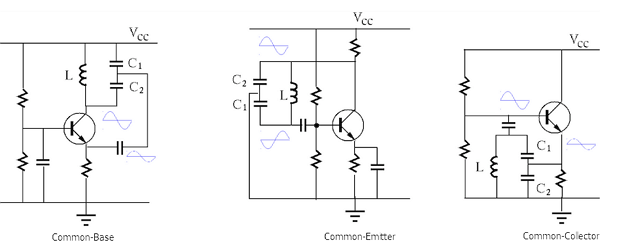# Quick Look at a Colpitts Oscillator

Quote of the Day

The most beautiful people we have known are those who have known defeat, known suffering, known struggle, known loss, and have found their way out of the depths. These persons have an appreciation, a sensitivity, and an understanding of life that fills them with compassion, gentleness, and a deep loving concern. Beautiful people do not just happen.

## Introduction

We have Memorial Day off from work and that can only mean one thing – time to work on some circuits for home projects. I have another inductive sensor project for which I want to generate a frequency that depends on the inductance value. The Colpitts oscillator is a good circuit for this type of application.

This is a quick note to document how I analyzed a circuit that I found on a tutorial and verified a statement made on the tutorial about the minimum gain required to startup the oscillation. I also derived an expression for the oscillation frequency.

## Background

There are excellent Colpitts oscillator references available:

## Analysis

### Node Labeling

Figure 2 shows how I labeled the circuit nodes for analysis. It also shows where I opened the feedback loop (i.e. at the output).

### Circuit Analysis

Figure 3 shows my circuit analysis.

### Derivation

In Figure 4, I derive expressions for (1) the oscillation frequency, and (2) the critical amplifier gain needed to ensure the circuit oscillates. The analysis process was straightforward:

• set VIN = V3
• set s = j·ω because I am only interested in the steady-state sinusoidal solution.
• set the imaginary part of the loop equation to zero and solve for the frequency at which the phase shift is zero – a required condition for oscillation. This will give me my oscillation frequency.
• set the real part of the loop equation equal to one and solve for R2 – this effectively sets the gain condition for oscillation. Since the gain is given by R2/R1, this will allow me to solve for the required gain in terms of R1. This gain will be a minimum,  and we need to exceed that gain slightly to ensure the startup of the oscillator.

I computed a minimum gain of ~2.7 and an oscillation frequency of 11. 7 kHz. My simulation (below) confirmed these results.

When people discuss Colpitts oscillators, they usually state that the oscillation frequency is given by the equation${{f}_{{Standard}}}\left( {{{C}_{1}},{{C}_{2}},L} \right)=\frac{1}{{2\cdot \pi }}\sqrt{{\frac{{{{C}_{1}}+{{C}_{2}}}}{{{{C}_{1}}\cdot {{C}_{2}}\cdot L}}}}$. This is not the equation I derive in Figure 4$\displaystyle \left( {{{f}_{{Mine}}}\left( {{{C}_{1}},{{C}_{2}},L} \right)=\frac{1}{{2\cdot \pi }}\sqrt{{\frac{{L+{{R}_{1}}\cdot {{R}_{3}}\cdot \left( {{{C}_{1}}+{{C}_{2}}} \right)}}{{{{R}_{1}}\cdot {{R}_{3}}\cdot {{C}_{1}}\cdot {{C}_{2}}\cdot L}}}}} \right)$. This is because most Colpitts discussions are about current source-based, transistor designs versus the voltage source-based, opamp design considered here. This difference in topology makes the circuit equations slightly different. I show in Figure 5 that when${{R}_{1}}\cdot {{R}_{3}}\cdot \left( {{{C}_{1}}+{{C}_{2}}} \right)>>L$ (usually the case), the standard Colpitts equation and my equation are approximately equal.

### Simulation

The nodes in my simulation are labeled differently than in my mathematical work above. LTSpice wants to name the nodes in a manner that it finds convenient.

#### LTSpice Circuit

Figure 6 shows my implementation of the circuit using LTSpice. To force the oscillator to start, I set node 2 = 0.3 V at startup with the Initial Condition command (IC) – in real circuits, noise and the power supply ramp on startup drives the oscillator into action.

#### Results

Figure 7 shows my simulation results. The oscillation frequency is slightly lower (11.5 kHz) than the mathematical analysis showed because the analysis ignored the opamp's frequency response – the phase shift of the amplifier contributes to the where the circuit oscillates.

While not shown here, I tried various gains, and I confirmed that I needed an amplifier gain of ~3 to get the oscillator to startup.Figure 7: Simulation Results. Observe that the sinusoid at node 4 is pretty clean compared to the amplifier output.

I should note that all oscillators have some level of distortion caused by their amplitude-limiting circuitry, which is intrinsically a nonlinear function. For example, Bill Hewlett used a lamp with a nonlinear resistance characteristic to stabilize the old HP200's amplitude level – as elegant a solution as I can imagine.

In this case, amplitude stability is maintained because of nonlinearities in the amplifier, which is NOT the way to achieve a quality sinusoid. However, I am not building a lab grade project here – purely a home project.

## Conclusion

I will be building this circuit this weekend. I am hopeful that the analysis and simulation matches reality. My first step is ALWAYS to get a valid mathematical analysis and working simulation before I build anything.

This entry was posted in Electronics. Bookmark the permalink.

### 5 Responses to Quick Look at a Colpitts Oscillator

1.Benson Niou says:

Could you do this in common collector amplifier ?

Thanks

•mathscinotes says:

You can build this oscillator using Common-Base, Common-Collector, and Common-Emitter. The figure below shows the basic configurations.There is a very good discussion of these configurations on this web page, where I got the figure above.

mark

2.Bhakti C. says:

Why have you considered a 200 ohms resistor between Vin and V1?